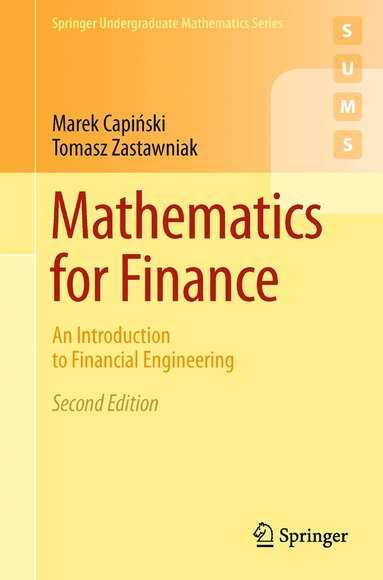Vetenskap & teknik

Mathematics for Finance: An Introduction to Financial Engineering

Marek Capinski•Tomasz Zastawniak

329:- (389:-)

Funktionen begränsas av dina webbläsarinställningar (t.ex. privat läge).

Hos dig inom 2-6 arbetsdagar

Fri frakt vid köp över 149:- för medlemmar.

• 336 sidor
• 2010

As with the first edition, Mathematics for Finance: An Introduction to Financial Engineering combines financial motivation with mathematical style. Assuming only basic knowledge of probability and calculus, it presents three major areas of mathematical finance, namely Option pricing based on the no-arbitrage principle in discrete and continuous time setting, Markowitz portfolio optimisation and Capital Asset Pricing Model, and basic stochastic interest rate models in discrete setting.

From the reviews of the first edition:

This text is an excellent introduction to Mathematical Finance. Armed with a knowledge of basic calculus and probability a student can use this book to learn about derivatives, interest rates and their term structure and portfolio management. (Zentralblatt MATH)

Given these basic tools, it is surprising how high a level of sophistication the authors achieve, covering such topics as arbitrage-free valuation, binomial trees, and risk-neutral valuation. (www.riskbook.com)

The reviewer can only congratulate the authors with successful completion of a difficult task of writing a useful textbook on a traditionally hard topic. (K. Borovkov, The Australian Mathematical Society Gazette, Vol. 31 (4), 2004)

• Författare: Marek Capinski, Tomasz Zastawniak